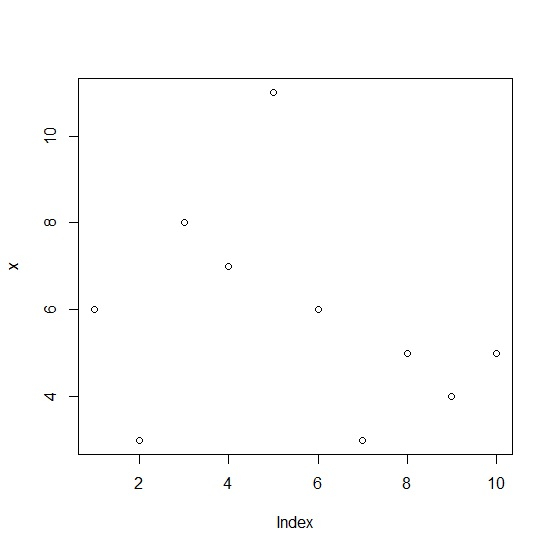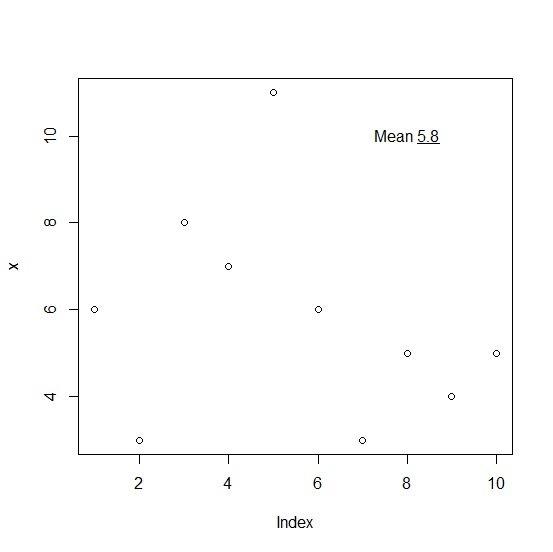# How to display mean with underline in base R plot?

To display mean value with underline in base R plot, we can use underline function within text function. The text function will be used to write the word Mean in the plot and underline function will underline the mean.

Check out the below example to understand how it works.

## Example

Following snippet creates a sample data frame −

x<-rpois(10,5)
x

## Output

The following dataframe is created −

 6 3 8 7 11 6 3 5 4 5


To display mean with underline in base R plot, add the following code to the above snippet −

x<-rpois(10,5)
plot(x)

## Output

If you execute all the above given codes as a single program, it generates the following output −To display mean with underline in base R plot, add the following code to the above snippet −

text(8,10,bquote("Mean"~underline(.(mean(x)))))


## Output

If you execute all the above given codes as a single program, it generates the following output −